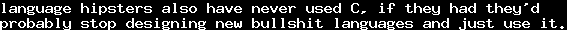# A short thought on the axiom of choiceThu, 11 Aug 2016 16:14:34 GMT

It is a well-known fact that:

Assume we wanted to prove. We can then, by the schema of specification, define the setsand. We constructively know that both sets are non-empty: At leastand. Therefore,is a set of non-empty sets, and therefore, by the axiom of choice, there is a functionsuch thatand, that is, we know thatand. Trivially,, hence,. Similarly,. Hence,. On the other hand,, therefore also. Contraposition (which is constructive in this direction) yields. Hence,.

Now, in my opinion, the problem here is not the axiom of choice. Let us recall what the axiom of choice says: If we proved that every set in a collection has an element, then we can find an element for every such set. I find this principle very constructive: If we can prove that something exists, then we can find it. As long as we cannot prove the existence of things that we cannot find, the axiom of choice should not do any harm to constructivity, as far as I see – correct me if I am wrong.

Of course, if you can prove the existence of things that you cannot find, the axiom of choice might make the situation worse. And in my opinion, in the case of ZFC, in this proof, the schema of specification is the actual culprit. Whcih is why I wonder why even some "constructive" set theories have a similar axiom scheme. For some reason, the impression that "smaller" sets are always simpler than "larger" sets is prevalent, and from that perspective, separation is not a problem. I wonder where this comes from.

Even without any deeper knowledge of recursion theory or logic, it should be clear that while the set of natural numbersis rather simple, there are subsets which are more complicated. Of course, you can encode the halting problem into a subset of, but you don't have to go that far: Consider the set of prime numbers. It is intuitively more complicated than. (This somehow reminds me of the concept of entropy, considering the "amount of information" encoded in a set. Not sure whether there is a formal correspondence, though.)

An obvious limitation one could impose to comprehension-like schemes would be that the propositions used need to be provably decidable. This would break the above proof in the sense thatwould be decidable from the start. And it makes more sense in a constructive setting: If we assume that we can decide a set, the resulting set would still be decidable. Not sure whether there exists a constructive set theory with such an axiom, though.

Just my two cent. Feel free to enlighten me if I am wrong.